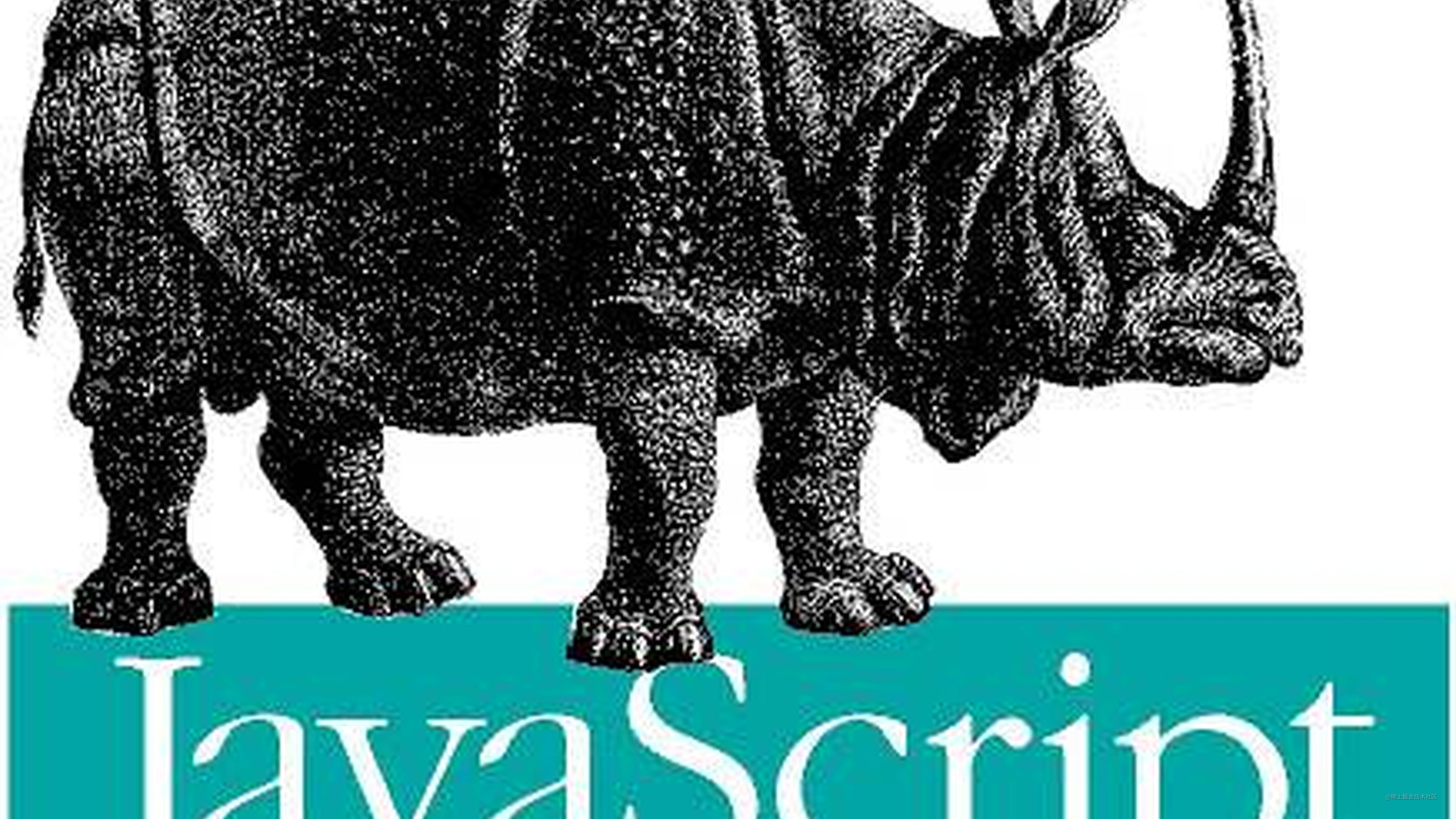# 0.1 加 0.2 为什么不等于 0.3？2021-02-10更新： 这篇文章我并非完全理解，有一些疏漏之处。我重写整理了思路，写了一篇，对浮点数机制进行了更完备的说明，传送门：0.1 加 0.2 不等于 0.3 ？从计算机角度深挖 JavaScript 浮点数存储机制

## 什么是浮点数？

### 计算机中数的表示

• 定点数：小数点位置确定。假设16位存储，小数点在符号位后面成为小数定点机（这类机器只能表示小数），小数点在末尾成为整数定点机（这类机器只能表示整数）。
• 浮点数表示类似于十进制的科学计数法表示。由阶数（阶码，阶符），尾数（尾码，尾符）构成。尾数的位数决定了浮点数的精度，阶数的位数决定了浮点数的范围。

### 浮点数的规格化

1. 对于小数来说，转换为二进制是乘 2 取整操作，例如将 0.1 转换成二进制

运算过程取整小数部分
0.1 * 2 = 0.200.2
0.2 * 2 = 0.400.4
0.4 * 2 = 0.800.8
0.8 * 4 = 1.610.6
0.6 * 2 = 1.210.2
.........
2. 基于 IEEE74 标准（见下面部分）的双精度浮点数（尾数最多 52 位），我们可以得到如下结果：

$0.1 = 0.0001\quad1001100110011001100110011001100110011001100110011001（52）$
1. 规格化处理
• 规格化定义（r 表示阶基值,这里为 2，S 表示尾数）

$0.1 = 0.0001\quad1001100110011001100110011001100110011001100110011001（52）$
• 对于负数形式的补码，规格化的定义不适用（下面负数补码表示-1，不满足规格化定义）

S>0规格化形式S<0规格化形式
真值0.1XX...X真值-0.1XX...X
原码0.1XX...X原码1.1XX...X
补码0.1XX...X补码1.0XX...X
反码0.1XX...X反码1.0XX...X
• 因此通常来说

对于原码，不论整数，负数，第一数位为 1 即为规格化

对于补码，符号位和第一数位不同为规格化

在计算机中我们通常使用异或电路，当符号位和第一数位不同时，表示规格化完成

### IEEE754 标准

• 数字存储格式:S（数符）+ 阶码（含阶符）+ 尾数
• 尾数为规格化表示
• 非“0”的有效位最高位为“1”（隐含）

### 0.1 和 0.2 在计算机中是如何存储的

\begin{aligned} 0.1 = 0.0001\quad&1001100110011001100110011001100110011001100110011001 （52）\\ 0.2 = 0.001\quad&1001100110011001100110011001100110011001100110011001 （52） \end{aligned}

\begin{aligned} S（0.1） = 1.10011001100...1 （1100\times12）\\ S（0.2） = 1.100110011...001 （0011\times12）\\ P（0.1） = 1,0000000100（-4）\\ P（0.2） = 1,0000000011（-3） \end{aligned}

\begin{aligned} &0.1 \Rightarrow 0:01111111100:1001100110011001100110011001100110011001100110011010\\ &0.2 \Rightarrow 0:01111111101:1001100110011001100110011001100110011001100110011010\\ \\ &0.1 = 2^{-4} \times .1001100110011001100110011001100110011001100110011010\\ &0.2 = 2^{-3} \times .1001100110011001100110011001100110011001100110011010 \end{aligned}

### 0.1+0.2 在计算机中是如何运算的

\begin{aligned} 0.1 = 2^{-3}\times&0.1100110011001100110011001100110011001100110011001101(0)\\ 0.2 = 2^{-3}\times&1.1001100110011001100110011001100110011001100110011010\\ sum = 2^{-3}\times1&0.0110011001100110011001100110011001100110011001100111\\ \end{aligned}

### IEEE754 标准浮点数舍入模型

IEEE754 标准对浮点数进行舍入时，一共定义了四种模型

Round to Nearest - roundTiesToEven （Default）: 向最近的数靠近，最近的数需满足最低有效位为 0 或者偶数

Round toward 0: 向 0 靠近

Round toward +∞: 向正无穷靠近

Round toward −∞: 向负无穷靠近

Mode / Example Value+11.5+12.5−11.5−12.5
to nearest, ties to even （默认模型）+12.0+12.0−12.0−12.0
toward 0+11.0+12.0−11.0−12.0
toward +∞+12.0+13.0−11.0−12.0
toward −∞+11.0+12.0−12.0−13.0
to nearest, ties away from zero+12.0+13.0−12.0−13.0

## 误差的产生

\begin{aligned} a = 2^{-2}\times&1.0011001100110011001100110011001100110011001100110011(0)\\ sum = 2^{-2}\times&1.0011001100110011001100110011001100110011001100110011(1)\\ b=2^{-2}\times&1.0011001100110011001100110011001100110011001100110100(0)\\ \end{aligned}

\begin{aligned} 0.1+0.2=2^{-2}\times&1.0011001100110011001100110011001100110011001100110100\\ 0.3 = 2^{-2}\times&1.0011001100110011001100110011001100110011001100110011\\ \\ 0.1 + 0.2 \Rightarrow 0:01111111101&:0011001100110011001100110011001100110011001100110\\ 0.3 \Rightarrow 0:01111111101&:0011001100110011001100110011001100110011001100110 \end{aligned}

\begin{aligned} 0.1 + 0.2 = &0.300000000000000044408920985006...\\ 0.3 = &0.299999999999999988897769753748... \end{aligned}

var ll = 0.300000000000000044408920985006; // 中间有15个0
var lll = 0.299999999999999988897769753748;

## 总结

• 一道涉及 JS 基础数据结构—浮点数的问题，深入探究起来，让自己又复习了一波计算机组成原理的知识。

• 当时学习计组的时候，书上对 IEEE754 标准说得也很少，甚至不知道 IEEE754 的舍入标准。以至于之前的笔记我都认为 sum 的舍入使用的是学过的 0 舍 1 入法。直到写下这篇文章，揭开 IEEE 754 的面纱，才发现没那么简单。

## 参考

1. [Is floating point math broken? -- stack overflow（answer by Wai Ha Lee）]（stackoverflow.com/a/28679423）

2. [Rounding floating-point numbers -- Wikipedia]（en.wikipedia.org/wiki/IEEE_7…

3. [IEEE 754: Rounding Rules -- Wikipedia]（en.wikipedia.org/wiki/IEEE_7…